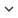ENGArticle
Article 304
Author/s
• Ismael Rodriguez-Fdez, Manuel Mucientes, Alberto Bugarin
DOI
Source
• Knowledge-Based Systems, 2016 - Q1

# S-FRULER: Scalable Fuzzy Rule Learning through Evolution for Regression

In genetic fuzzy systems (GFS) the size of the problem has a huge influence in the performance of the obtained models, since i) the fuzzy rule bases learned suffer from exponential rule explosion when the number of variables increases, and ii) the convergence time increments with the number of examples. In this paper we present S-FRULER, a scalable distributed version of FRULER which is a GFS that learns simple and linguistic TSK-1 knowledge bases for regression problems. S-FRULER obtains models with high accuracy and low complexity, whilst reducing the algorithm runtime. S-FRULER focuses on splitting the problem into smaller partitions and incorporates a feature selection process for reducing the number of variables used in each partition. Each partition is then solved independently using the FRULER algorithm. Afterwards, an aggregation function obtains the final linguistic TSK fuzzy rule base from the information generated in each partition. S-FRULER has been validated in terms of scalability, precision and complexity using 10 large-scale datasets and has been compared with three state of the art GFSs. Experimental results show that S-FRULER scales well while achieving simple models with a linguistic approach and a precision comparable with approximative models. Moreover, S-FRULER has been applied to a bioinformatics problem that was proposed as a benchmark for scalability of regression problems, obtaining good results in both accuracy and complexity.
Keywords: Genetic Fuzzy Systems, regression, instances selection, multi-granularity fuzzy discretization